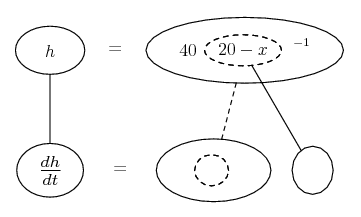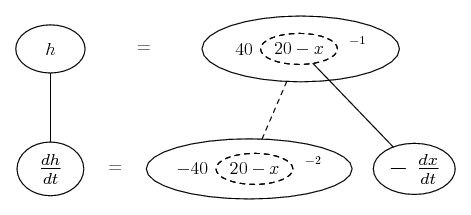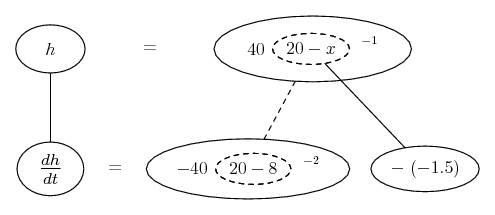# Thread: Relative Rates

1. ## Relative Rates

A spotlight is on the ground 20m away from a wall and a 2m tall person is walking towards the wall at a rate of 1.5m/s . How fast is the height of the shadow changing when the person is 8m from the wall? Is the shadow increasing or decreasing in height at this time?

i know i need to find dh/dt, which equals dx/dt * dh/x.

Is it possible to find dh/dx? (ie the relation between height of shadow and distance from wall)

2.Originally Posted by anonymous_mathsIs it possible to find dh/dx? (ie the relation between height of shadow and distance from wall)

Yes. Use the similar triangles in your sketch (?!) to say...

$\displaystyle \frac{h}{20} = \frac{2}{20 - x}$

Just in case a picture helps with the chain rule...... where... is the chain rule. Straight continuous lines differentiate downwards (integrate up) with respect to t, and the straight dashed line similarly but with respect to the dashed balloon expression (the inner function of the composite which is subject to the chain rule).

Spoiler:Spoiler:_________________________________________

Don't integrate - balloontegrate!

Balloon Calculus; standard integrals, derivatives and methods

Balloon Calculus Drawing with LaTeX and Asymptote!

3. Hello, anonymous_maths!

A spotlight is on the ground 20m away from a wall.
A 2m tall person is walking towards the wall at a rate of 1.5m/s.

How fast is the height of the shadow changing when the person is 8m from the wall?
Is the shadow increasing or decreasing in height at this time?
Code:
      |
S o
: |  *
: |     *
: |        *  P
h |           o
: |           |  *
: |           |2    *
: |           |        *
T o-----------o-----------o L
:     x     Q    20-x   :
: - - - -  20 - - - - - :

The light is at $\displaystyle L\!:\;\;TL = 20$

The person is: .$\displaystyle PQ = 2$
His distance from the wall is: .$\displaystyle x \:=\:TQ \quad\Rightarrow\quad QL \:=\:20-x$
This distance is decreasing at: .$\displaystyle \frac{dx}{dt} \:=\:\text{-}\frac{3}{2}$ m/sec.

The height of his shadow is: .$\displaystyle h = ST.$

From the similar right triangles, we have:

. . $\displaystyle \frac{h}{20} \:=\:\frac{2}{20-x} \quad\Rightarrow\quad h \:=\:40(20-x)^{-1}$

Differentiate with respect to time: .$\displaystyle \frac{dh}{dt} \;=\;\text{-}40(2-x)^{-2}(\text{-}1)\frac{dx}{dt} \;=\;\frac{40}{(20-x)^2}\frac{dx}{dt}$

When $\displaystyle x = 8\!:\;\;\frac{dh}{dt} \;=\;\frac{40}{12^2}\left(\text{-}\frac{3}{2}\right) \;=\;-\frac{5}{12}$

The height of the shadow is decreasing at $\displaystyle \tfrac{5}{12}$ meters per second.

#### Search Tags

calculas, derivative, differentiation, mathematics, rates, relative